Published: 15 February 2017

# Nonlinear dynamic modeling and fuzzy sliding-mode controlling of electromagnetic levitation system of low-speed maglev train

Yougang Sun1
Wanli Li2
Junqi Xu3
Haiyan Qiang4
Chen Chen5
1, 2, 4School of mechanical engineering, Tongji University, Shanghai, China
3National Maglev Transportation Engineering R&D Center, Tongji University, 201804, China
5, 1, 4College of Logistics Engineering, Shanghai Maritime University, Shanghai, China
Corresponding Author:
Yougang Sun
Views 617

#### Abstract

The electromagnet levitation system (ELS) of low-speed maglev train is taken as the research object. The nonlinear dynamics and control law of ELS are discussed. Specifically, by employing the Euler-Lagrange’s method, a nonlinear dynamic model is constructed for the single-ELS. Then, the linear control law is studied, which has a disadvantage of weak robustness. To improve the performance of the controller, a fuzzy sliding-mode control law is proposed. According to the dynamic nonlinear model, a novel sliding surface which can make the system reach the stable point within the finite time is presented. Moreover, the fuzzy inference method is utilized to slow down the speed of the states crossing the sliding surface. The simulation results demonstrate that the global robustness of external disturbance and parameter perturbation can be achieved through the proposed control law. And the chattering phenomenon can be reduced significantly. Finally, the experiments are also implemented to examine its practical dynamic performance of the proposed control law.

## 1. Introduction

The low-speed maglev transportation has already become a novel urban rail traffic owing to its excellent ability of turning and climbing, lower noise, investment and maintenance [1, 2]. HSST railway system with a top speed of 100 km/h, which is applied on Japan Nagoya Hill line, is a typical delegacy of low-speed maglev. In China, the first low-speed maglev business line had come into service in Changsha .

The core technology of low-speed maglev train is ELS which is known as electromagnetic levitation system. In recent years, tremendous attentions have been paid on levitation control which has already become a hotspot in gradual. Linear expansion at the balance point is a traditional levitation control method of maglev train. To design the controllers, linear control theories are used [4-7]. Nevertheless, when a large disturbance exists, the typical nonlinear system ELS will become unstable and be away from the balance point. The levitation airgap of maglev train is around 9 mm. Under the high-speed conditions, the instability will lead to fatal accidents. Because of the boarding and alighting passengers who will have immediate impact on the position of the balance point, the capacity of maglev train is so limited. Hence, to compare the traditional levitation control law to the modern levitation controller, the traditional one only paid emphasis on stability while the modern one not only paid attention to stability but also to the perfection of whole performance for the system, just like robustness, dynamic response quality etc. The researchers have proposed variety of intelligent control strategies for the ELS. Ginoya et al.  presented a state-and-disturbance-observer-based cascade sliding mode control for magnetic levitation system, which is robust to uncertainties and the t trajectory to be tracked. Xu et al.  presented a robust control method to guarantee the magnitude limitation of the airgap for the maglev vehicle, regardless of the uncertainty. Li et al.  proposed a novel conception of the virtual electromagnetic energy harvester (EEH) to emulate a real energy harvester, which can actively control the stationary self-excited vibration of the maglev system. Wai et al.  presented a self-adapted fuzzy-neural network control law for magnetic levitation system with higher control accuracy. Wang et al.  took rail flexible vibration as excitation, then use gain table control method in accordance with system quality automatic adjusting feedback gain parameter. But the nonlinear model of ELS was linearized when design the controller, and the problems of external disturbance and parameters perturbation were not taken into account. Su et al.  proposed a fuzzy control method based on nonlinear maglev system T-S (Takagi-Sugeno) fuzzy model. The simulations illustrated the method’s effectiveness dealing with bounded disturbance. However, the control performance was not verified in a real experiment. Wang et al.  designed state feedback controller by using the state estimator of Kaman filter which solved the levitation control system’s relay on rail beam stiffness. But the control plant used linear approximate mathematic model. When the system is far away from the balance point with external disturbance, the control performance will be reduced . Moreover, the parameter uncertainties were not mentioned.

Due to the aforementioned facts, the control schemes of ELS have made much progress during the past two decades, while there are still a number of problems in practice. For example, the maglev trains are easily affected by external disturbances of wind, step of rail and so on. In addition, there are also mismatches between theoretical model parameters and actual system parameter, including multiple parameters perturbations, such as the weight of passengers, inductance and resistance. However, the present control approaches either linearize the nonlinear dynamic model of the ELS or neglect some nonlinear term in the closed-loop systems. Those processing will reduce the dynamic performance of the controller in practice. If processing is improperly, it is most likely that the systems become instable. To address these problems, a fuzzy-sliding-mode-based robust control scheme is put forward for maglev train to against nonlinear disturbances and parameter perturbations. Specifically, this paper firstly establishes a nonlinear mathematical equation by the Euler-Lagrange method for single-ELS of maglev train. Then, the nonlinear model of ELS is transformed and a new manifold is constructed. Next, based on the manifold, a sliding mode controller is designed using exponential reaching law, which can ensure the system state in the manifold all the time. In order to reduce the chattering phenomenon, the fuzzy inference method is utilized to soften the switch control according to the switch states of sliding-mode. Sufficient simulation results are given to illustrate that the proposed controller can achieve better dynamic performance and effectively weaken the chattering of sliding-mode control. Finally, the new nonlinear controller is applied to the full-scale low-speed maglev train and the experimental results verify that this method shows a strong robustness to the external disturbance. Meanwhile, the energy consumption of the levitation system is much lower.

## 2. Dynamics development and analysis of ELS

ELS which is known as electromagnetic levitation system is used by the low-speed maglev train. The mature module control concept is utilized in the levitation chassis of the maglev train, which can realize the multiple electromagnets decoupling [16-18]. Thus, the multiple electromagnets levitation control system is deformalized into a single-electromagnet control system as shown in Fig. 1.

The train body of the maglev train is supported by the air springs. The load-force is transmitted to each single-electromagnet through the levitation chassis. Because the stiffness of air spring supporting train body is less than that of the levitation stiffness, the electromagnet’s load variation caused by slight vibration of train body can be neglected . Therefore, the levitation chassis and train body are commonly considered as a whole [4, 11, 12, 19, 20]. $m$ denotes the mass of train body and levitation chassis. ${x}_{m}\left(t\right)$ represents the airgap between the rail and electromagnet. ${x}_{ref}$ and $\mathrm{\Delta }x$denote desired airgap and airgap error. ${N}_{m}$ is the number of turns in the electromagnetic coil. ${A}_{m}$ denotes pole area of the electromagnet; ${u}_{m}\left(t\right)$ and ${i}_{m}\left(t\right)$ represent the voltage and current in electromagnetic coil; ${L}_{M}\left({x}_{m}\left(t\right)\right)$ and ${R}_{m}$ denote the inductance and the resistance of the electromagnetic coil, respectively.

Fig. 1Configuration of the electromagnetic levitation system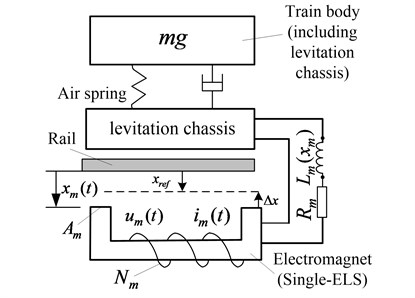We will utilize the Euler-Lagrange equation to derivate the single-ELS dynamics mathematical model. The equation of Euler-Lagrange can be expressed as follows:

1
$\frac{d}{dt}\left(\frac{\partial \left(T-V\right)}{\partial {\stackrel{˙}{q}}_{i}}\right)-\frac{\partial \left(T-V\right)}{\partial {q}_{i}}={Q}_{i},$

where, $T$ is kinetic energy and $V$ is potential energy. ${q}_{i}$, ${\stackrel{˙}{q}}_{i}$ and ${Q}_{i}$ are generalized coordinate, velocity and force, respectively.

The generalized coordinate and velocities are selected as ${q}_{1}={x}_{m}\left(t\right)\text{,}$${\stackrel{˙}{q}}_{1}={\stackrel{˙}{x}}_{m}\left(t\right)\text{,}$${\stackrel{˙}{q}}_{2}={i}_{m}\left(t\right)$. The kinetic energy and potential energy of system can be written as follows:

2
$T=\frac{1}{2}{L}_{M}\left({x}_{m}\left(t\right)\right){i}_{m}^{2}\left(t\right)+\frac{1}{2}m{\stackrel{˙}{x}}_{m}^{2}\left(t\right),$
3
$V=-mg{x}_{m}\left(t\right).$

The Lagrangian can be derived as follows:

4
$L=\frac{1}{2}{L}_{M}\left({x}_{m}\left(t\right)\right){i}_{m}^{2}\left(t\right)+\frac{1}{2}m{\stackrel{˙}{x}}_{m}^{2}\left(t\right)+mg{x}_{m}\left(t\right),$

where, ${L}_{M}\left({x}_{m}\left(t\right)\right)$ denotes the inductance of the electromagnet, which is a nonlinear function about the airgap ${x}_{m}\left(t\right)$. Ignoring leakage flux, ${L}_{M}$ can be expressed as below:

5
${L}_{M}\left({x}_{m}\left(t\right)\right)=\frac{{\mu }_{0}{N}_{m}^{2}{A}_{m}}{2{x}_{m}\left(t\right)}.$

The generalized forces of ELS are ${Q}_{1}={f}_{d}$ and ${Q}_{2}={u}_{m}\left(t\right)-{i}_{m}{R}_{m}$, respectively.

Therefore, from the general form of Lagrangian equation can be written as follows:

6
$\left\{\begin{array}{l}\frac{\partial L}{\partial {q}_{1}}=\frac{\partial L}{\partial {x}_{m}\left(t\right)}=-\frac{{\mu }_{0}{A}_{m}{N}_{m}^{2}{i}_{m}^{2}}{4{x}_{m}^{2}\left(t\right)}+mg,\\ \frac{\partial L}{\partial {\stackrel{˙}{q}}_{1}}=\frac{\partial L}{\partial {\stackrel{˙}{x}}_{m}\left(t\right)}=m{\stackrel{˙}{x}}_{m}\left(t\right),\\ \frac{d}{dt}\left(\frac{\partial L}{\partial {\stackrel{˙}{q}}_{1}}\right)=m{\stackrel{¨}{x}}_{m}\left(t\right),\\ \frac{d}{dt}\left(\frac{\partial L}{\partial {\stackrel{˙}{q}}_{1}}\right)-\frac{\partial L}{\partial {q}_{1}}={f}_{d},\\ {\stackrel{¨}{x}}_{m}\left(t\right)=\frac{{f}_{d}}{m}+g-\frac{{\mu }_{0}{A}_{m}{N}_{m}^{2}{i}_{m}^{2}}{4m{x}_{m}^{2}\left(t\right)},\end{array}\right\$
7
$\left\{\begin{array}{l}\frac{\partial L}{\partial {q}_{2}}=0,\\ \frac{\partial L}{\partial {\stackrel{˙}{q}}_{2}}=\frac{\partial L}{\partial {i}_{m}\left(t\right)}={L}_{M}\left({x}_{m}\left(t\right)\right){i}_{m}\left(t\right),\\ \frac{d}{dt}\left(\frac{\partial L}{\partial {\stackrel{˙}{q}}_{2}}\right)=-\frac{{\mu }_{0}{A}_{m}{N}_{m}^{2}{i}_{m}}{2{x}_{m}^{2}}{\stackrel{˙}{x}}_{m}+{L}_{M}\frac{d{i}_{m}}{dt},\\ \frac{d}{dt}\left(\frac{\partial L}{\partial {\stackrel{˙}{q}}_{2}}\right)-\frac{\partial L}{\partial {q}_{2}}={u}_{m}-{i}_{m}{R}_{m},\\ \frac{d{i}_{m}}{dt}=\frac{2{x}_{m}\left(t\right){u}_{m}}{{\mu }_{0}{N}_{m}^{2}{A}_{m}}-\frac{2{x}_{m}{i}_{m}{R}_{m}}{{\mu }_{0}{N}_{m}^{2}{A}_{m}}+\frac{{i}_{m}}{{x}_{m}^{}}{\stackrel{˙}{x}}_{m}.\end{array}\right\$

System states are selected as ${y}_{1}\left(t\right)={x}_{m}\left(t\right)$, ${y}_{2}\left(t\right)={\stackrel{˙}{x}}_{m}\left(t\right)$ and ${y}_{3}\left(t\right)={i}_{m}\left(t\right)$. The state space expressions of ELS can be written as below:

8
$\left\{\begin{array}{l}{\stackrel{˙}{y}}_{1}\left(t\right)={y}_{2}\left(t\right),\\ {\stackrel{˙}{y}}_{2}\left(t\right)=-\frac{{\mu }_{0}{A}_{m}{N}_{m}^{2}}{4m}{\left[\frac{{y}_{3}\left(t\right)}{{y}_{1}\left(t\right)}\right]}^{2}+g+\frac{1}{m}{f}_{d},\\ {\stackrel{˙}{y}}_{3}\left(t\right)=\frac{{y}_{2}\left(t\right){y}_{3}\left(t\right)}{{y}_{1}\left(t\right)}+\frac{2{y}_{1}\left(t\right)}{{\mu }_{0}{N}_{m}^{2}{A}_{m}}\left({u}_{m}\left(t\right)-{y}_{3}\left(t\right){R}_{m}\right).\end{array}\right\$

The levitation electromagnet and track are assumed to be located with a steady location, which is so called equilibrium state [21, 22], with the effect of certain loads and self-gravity. Meanwhile, levitation airgap of magnet is ${x}_{ref}$ and the equilibrium voltage is ${u}_{me}$. The levitation system works at the balanced position${y}_{1e}={x}_{ref}$ in usual, while ${y}_{e}\triangleq \left({y}_{1e},{y}_{2e},{y}_{3e}{\right)}^{T}=\left({x}_{ref},0,\sqrt{\kappa }{x}_{ref}{\right)}^{T}$ and ${u}_{me}=\sqrt{\kappa }{R}_{m}{x}_{ref}$are chosen as the equilibrium state, where, $\kappa \triangleq 4mg/{\mu }_{0}{N}_{m}^{2}{A}_{m}$.

To simplify the following system analysis, the following coordinate transformation can be introduced:

9
$z\left(t\right)\triangleq \left[\begin{array}{l}{z}_{1}\left(t\right)\\ {z}_{2}\left(t\right)\\ {z}_{3}\left(t\right)\end{array}\right]=\left[\begin{array}{c}{y}_{1}\left(t\right)-{y}_{1e}\\ {y}_{2}\left(t\right)\\ {y}_{3}\left(t\right)-{y}_{3e}\end{array}\right].$

$\upsilon \left(t\right)={u}_{m}\left(t\right)-{u}_{me}$ is defined and the coordinate transformation above is adopted. The system state space expressions which are equivalent to Eq. (8) can be rewritten as:

10
$\left\{\begin{array}{l}{\stackrel{˙}{z}}_{1}\left(t\right)={z}_{2}\left(t\right),\\ {\stackrel{˙}{z}}_{2}\left(t\right)=\frac{\left(2{x}_{ref}+{z}_{1}\left(t\right)\right){z}_{1}\left(t\right)g}{\left({z}_{1}\left(t\right)+{x}_{ref}{\right)}^{2}}-\frac{\left(2\sqrt{\kappa }{x}_{ref}+{z}_{3}\left(t\right)\right){z}_{3}\left(t\right)g}{\kappa \left({z}_{1}\left(t\right)+{x}_{ref}{\right)}^{2}}+\frac{1}{m}{f}_{d},\\ {\stackrel{˙}{z}}_{3}\left(t\right)=\frac{{z}_{3}\left(t\right)+\sqrt{\kappa }{x}_{ref}}{{z}_{1}\left(t\right)+{x}_{ref}}{z}_{2}\left(t\right)+\frac{\kappa \left({y}_{1}\left(t\right)+{x}_{ref}\right)}{2mg}\left(\upsilon \left(t\right)-{z}_{3}\left(t\right){R}_{m}\right).\end{array}\right\$

It is apparent that the state space model Eq. (10) of levitation system is strongly nonlinear. The parameters of levitation system are listed in Table 1.

Table 1Parameters values of the levitation system

 Physical quantity Value Physical quantity Value Mass $m$ / kg 700 Leakage permeance $\eta$ 0 Number of turns of coil ${N}_{m}$ 450 ${\mu }_{0}$ / (H·m-1) 4$\pi$×10-7 Area of coil ${A}_{m}$ / m2 0.024 Stable current ${i}_{ref}$ / A 19.1 Coil resistance ${R}_{m}$ / Ω 1.2 Stable airgap ${x}_{ref}$ / m 0.009

## 3. Control strategy design and simulations

### 3.1. Nonlinear sliding mode controller

Since the conventional linear control algorithm cannot reject the nonlinear dynamic disturbance and multi-parameters perturbations/uncertainties satisfactorily, the number of passengers in low-speed maglev train is limited strictly. In order to solve this problem, a continuous robust nonlinear control algorithm is developed in this section. As is known to all, the sliding mode is a robust control method and can effectively deal with parameter perturbations and disturbance. But for the strong nonlinear systems such as ELS, it is difficult to design the control algorithm without any linear approximation and analyze the stability on the sliding mode surface. We will design and modify the sliding mode controller based on nonlinear mathematic model without any approximation subsequently.

The state variables of system are chosen as follows:

11
${x}_{1}={x}_{m}\left(t\right),$
12
${x}_{2}={\stackrel{˙}{x}}_{1}={\stackrel{˙}{x}}_{m}\left(t\right).$

The airgap error and error rate are defined in the following manner:

13
$e=r-{x}_{1},$
14
$\stackrel{˙}{e}=\stackrel{˙}{r}-{\stackrel{˙}{x}}_{1}=\stackrel{˙}{r}-{x}_{2},$

where, $r$ is the target levitation airgap.

The sliding mode surface of the system is designed as:

15
$S=CE=C\left(R-X\right),$

where, $C=\left[{c}_{1},{c}_{2}\right]$, $R=\left[r,\stackrel{˙}{r}{\right]}^{T}$, $X=\left[{x}_{1},{x}_{2}{\right]}^{T}$.

To ensure the stability of the system, the Hurwitz’s stability need to be satisfied, which is ${c}_{1}/{c}_{2}>0$.

It must meet the requirement that $\underset{s\to 0}{\mathrm{l}\mathrm{i}\mathrm{m}}sds/dt\le 0$ to guarantee the existence and reachability of the sliding mode. The moving point’s stability condition on the sliding surface is $ds/dt=0$.

The following equation should be satisfied with the system’s entrance into sliding motion:

16
$\stackrel{˙}{S}={c}_{1}\left(\stackrel{˙}{r}-{\stackrel{˙}{x}}_{1}\right)+{c}_{2}\left(\stackrel{¨}{r}-\frac{{P}_{x}{x}_{1}}{m}+\frac{{P}_{i}{u}_{s}}{m}-\frac{{f}_{d}}{m}\right),$

where, ${u}_{s}$denotes the output of the controller. ${f}_{d}$ is disturbance, and $\left|{f}_{d}\right|\le D$. $D$ is the maximum upper bound of disturbance.

The controller is designed by the exponential reaching law which is shown as follow:

17
$\stackrel{˙}{S}=-\xi \mathrm{s}\mathrm{g}\mathrm{n}\left(S\right)-kS,$

where, $\xi$ and $k$ denote constant reaching coefficient and exponential reaching coefficient, respectively.

Based on (13), (14) and (17), the sliding mode controller (SMC) of the system can be designed as follow:

18
${u}_{s}=\frac{m}{{c}_{2}{P}_{i}}\left(-\xi \mathrm{s}\mathrm{g}\mathrm{n}\left(S\right)-kS-{c}_{1}\stackrel{˙}{r}+{c}_{1}{\stackrel{˙}{x}}_{1}-{c}_{2}\stackrel{¨}{r}+\frac{{c}_{2}{P}_{x}{x}_{1}}{m}+\frac{{c}_{2}D\mathrm{s}\mathrm{g}\mathrm{n}\left(S\right)}{m}\right).$

Stability analysis:

Lyapunov function candidate is selected as:

19
$V\left(x\right)=\frac{1}{2}{S}^{2}.$

This function is positive semi-definite, and the derivative of the both side can be obtained as:

20
$\stackrel{˙}{V}\left(x\right)=S\cdot \stackrel{˙}{S}=-S\cdot \xi \mathrm{s}\mathrm{g}\mathrm{n}\left(S\right)-k\cdot {S}^{2}=-\xi \left|S\right|-k\cdot {S}^{2}\le 0.$

Based on the Lyapunov theorem, it is easy to prove that the system is global asymptotically stable.

The sliding mode controller (SMC) is designed in the form of (18). Set ${c}_{1}=$ 8, ${c}_{2}=$ 1, $k=$ 7, $\xi =$ 13. The target airgap is 9 mm. The initial position is 17 mm. In order to demonstrate the increased performance over traditional linear controller, we compare the proposed controller with PID controller. The parameters of PID controller are sufficiently tuned to obtain the best performance, which yields the following values: ${K}_{P}=$ 5700, ${K}_{I}=$ 1700, ${K}_{D}=$ 2400.

The simulation results of airgap with PID controller and FSMC controller are illustrated in Fig. 2. The simulation results of control input are shown in Figs. 3. The airgap response with PID controller has oscillation. The SMC has better dynamic performance than PID, and has no overshoot. The response of levitation airgap of SMC is faster than PID controller and stability performance of SMC are better. But the control current of SMC as shown in Fig. 3 shows that high frequency oscillations exist in the controller inputs, which is called chattering phenomenon.

Fig. 2Simulation results of airgap (mm)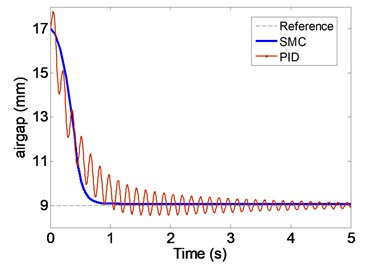Fig. 3Simulation results of control current (SMC)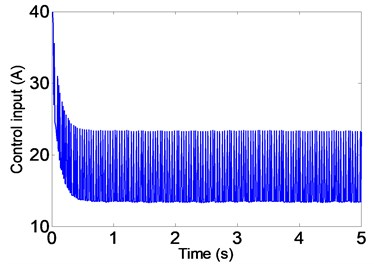The dynamic disturbance shown in Fig. 4 is applied to the system. The simulation results of airgap under disturbance are shown in Figs. 5. The control current of SMC under the dynamic disturbance is shown in Fig. 6.

Fig. 4Periodic dynamic disturbance force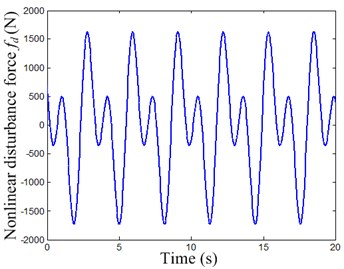Fig. 5Airgap under disturbance (mm)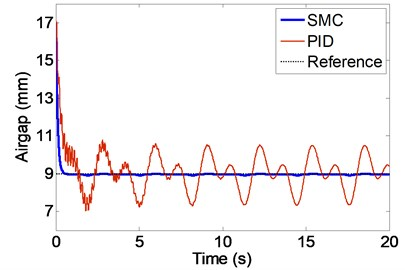Fig. 6Control current under disturbance (SMC)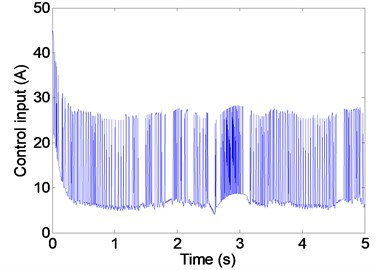We can learn from the Fig. 5 that the system has no overshoot and the regulation is completed within 0.5 s. Comparing PID control performance, the SMC has stronger robustness against disturbance. However, Figs. 6 illustrates that the chattering phenomenon exist in the controller inputs. The chattering will bring mechanical wear to the actuator and increase the consumption of energy, which restrict the application of sliding mode control in the engineering practice .

### 3.2. Fuzzy-sliding mode controller

To reduce the chattering phenomenon, a fuzzy inference method is utilized to improve the sliding mode controller.

The sliding mode control strategy is expressed as follows:

21
${u}_{s}=\frac{m}{{c}_{2}{P}_{i}}\left(-kS-{c}_{1}\stackrel{˙}{r}+{c}_{1}{\stackrel{˙}{x}}_{1}-{c}_{2}\stackrel{¨}{r}+\frac{{c}_{2}{P}_{x}{x}_{1}}{m}+\frac{{c}_{2}D\mathrm{s}\mathrm{g}\mathrm{n}\left(S\right)}{m}-\xi \mathrm{s}\mathrm{g}\mathrm{n}\left(S\right)\right)={u}_{eq}+{u}_{sw},$

where, the switching control law is ${u}_{sw}=\frac{m}{{c}_{2}{P}_{i}}\left({c}_{2}D\mathrm{s}\mathrm{g}\mathrm{n}\left(S\right)/m-\xi \mathrm{s}\mathrm{g}\mathrm{n}\left(S\right)\right)$.

The switching control law ${u}_{sw}$ contains the symbol term$\mathrm{s}\mathrm{g}\mathrm{n}\left(\cdot \right)$, which leads to the sliding mode variable structure control cannot keep the control object on the sliding surface. The control object crosses back and forth on both ends of the sliding surface to generate chattering. In order to suppress the chattering and the influence of disturbance, fuzzy control is introduced to the control law [23-26]. The output of the fuzzy control is a new switched control law ${u}_{f}$, which instead of the gain term in the switching control of the traditional sliding mode control. The control signal is smoothed by the fuzzy control, thereby reducing the chattering. To sum up, the control diagram of ELS based on variable structure control strategy with fuzzy sliding mode can be illustrated in Fig. 7.

Fig. 7Control block diagram of FSMC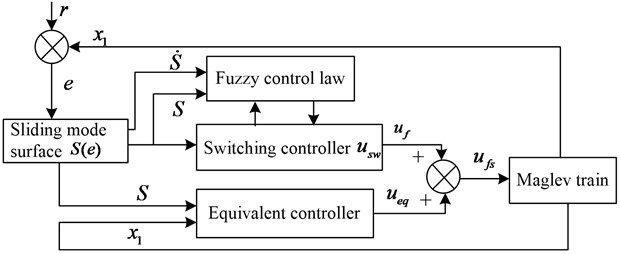${u}_{f}$ is the output of a fuzzy controller. After adding fuzzy control, the inputs of fuzzy control are $S$ and $\stackrel{˙}{S}$. $S$ represents the distance between the phase locus and the sliding surface of the system, while $\stackrel{˙}{S}$ is expressed as the velocity of the sliding surface. The unmodeled high-frequency of the system and other disturbance will be indirectly reflected in the $\stackrel{˙}{S}$ through ${\stackrel{˙}{x}}_{1}$, so it is reasonable to use $S$ and $\stackrel{˙}{S}$ as the input of fuzzy controller to infer the value of ${u}_{sw}$.

In addition, the fuzzy processing method can filter out the high-frequency noise caused by internal disturbance and inaccurate measurement. The basic universe of discourse of control inputs $S\text{,}$$\stackrel{˙}{S}$ and output ${u}_{sw}$ in the two-dimensional fuzzy controller are: $\left[\begin{array}{ll}-\left|{S}_{\mathrm{m}\mathrm{a}\mathrm{x}}\right|& \left|{S}_{\mathrm{m}\mathrm{a}\mathrm{x}}\right|\end{array}\right]\text{,}$$\left[\begin{array}{ll}-\left|D{S}_{\mathrm{m}\mathrm{a}\mathrm{x}}\right|& \left|D{S}_{\mathrm{m}\mathrm{a}\mathrm{x}}\right|\end{array}\right]$, $\left[\begin{array}{ll}-\left|{{u}_{sw}}_{\mathrm{m}\mathrm{a}\mathrm{x}}\right|& \left|{{u}_{sw}}_{\mathrm{m}\mathrm{a}\mathrm{x}}\right|\end{array}\right]$, respectively. The linguistic variable of $S$, $\stackrel{˙}{S}$ and ${u}_{sw}$ are $S$, $DS$ and $UF$. The fuzzy universe of discourse is divided into 7 subsets: PB, PM, PS, ZO, NS, NM, NB. The grades of universe of discourse are expressed as follows: $S=$ {–6, –4, –2, 0, 2, 4, 6}, $DS=${–3, –2, –1, 0, 1, 2, 3}, $UF=$ {–6, –4, –2, 0, 2, 4, 6}.

The membership functions of each fuzzy linguistic value subsets are determined by triangle distribution as shown in Figs. 8-10.

Fig. 8Membership function of input S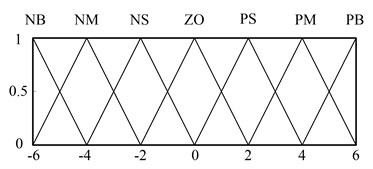Fig. 9Membership function of input S˙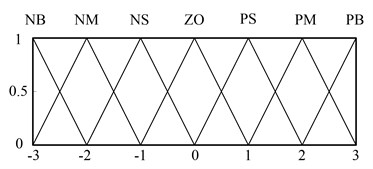Fig. 10Membership function of output usw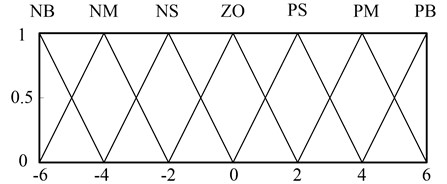The fuzzy rule for the switching control law is shown as follows:

${R}^{i}$: ($S\left(t\right)$ is ${A}^{i}$) and ($\stackrel{˙}{S}\left(t\right)$ is ${B}^{i}$) then (${u}_{sw}\left(t\right)$ is ${C}^{i}$), where $i=1,\dots ,n$, $n$ denotes the rule number. ${A}^{i}$, ${B}^{i}$ and ${C}^{i}$ represent fuzzy sets of $S\left(t\right)$, $\stackrel{˙}{S}\left(t\right)$ and ${u}_{sw}\left(t\right)$, respectively. Since each input has seven fuzzy sets, the total rule number is $N=$49 fuzzy rules. Table 2 describes the fuzzy control rules for the ELS.

In order to meet the conditions of $S\stackrel{˙}{S}<0$, the fuzzy control schemes for ${u}_{sw}\left(t\right)$ is described in Table 2.

Table 2Fuzzy rule for ELS

 NB NM NS ZO PS PM PB PB ZO PS PM PB PB PB PB PM NS ZO PS PM PB PB PB PS NM NS ZO PS PM PB PB ZO NB NM NS ZO PS PM PB NS NB NM NS ZO PS PM PB NM NB NB NM NS ZO PS PM NB NB NB NB NB NM NS ZO

Stability analysis:

Lyapunov function candidate is selected as:

22
$V\left(x\right)=\frac{1}{2}{S}^{2}.$

This function is positive semi-definite, and the derivative of the both side can be obtained as:

23
$\stackrel{˙}{V}\left(x\right)=S\cdot \stackrel{˙}{S}.$

It can be seen from the Table 2, when $s=PB$ and $\stackrel{˙}{s}=PB$, $s\stackrel{˙}{s}$ is also the same as PB. At the moment, it is necessary to control the input of positive and big variable to reduce $s\stackrel{˙}{s}$ rapidly and close to $s\stackrel{˙}{s}<0$. When $s$and $\stackrel{˙}{s}$were positive and negative respectively, it satisfies this condition of $s\stackrel{˙}{s}<0$. Thus, at this time the control variable is zero (ZO). When $s=NB$ and $\stackrel{˙}{s}=NB$, $s\stackrel{˙}{s}$ is also the same as PB. At this point, $s\stackrel{˙}{s}>0$ is positive and big. It is necessary to control the input of negative and big variable to reduce $s\stackrel{˙}{s}$ rapidly and close to $s\stackrel{˙}{s}<0$.

The fuzzy control system is stable because of all the control rules are designed based on $s\stackrel{˙}{s}<0$. We can obtain the conclusion as follows:

24
$\stackrel{˙}{V}\left(x\right)\le 0.$

Thus, $V\left(x\right)\ge 0$, $\stackrel{˙}{V}\left(x\right)\le 0$. Based on the Lyapunov theorem, it is easy to prove that the system is global asymptotically stable.

${c}_{1}$, ${c}_{2}$, $k$ and $\xi$ remain the same. The target airgap is 9 mm. The initial position is 17 mm. The system simulation results of position tracking performance are shown in Figs. 11-12. The dynamic disturbance of Fig. 4 is added to the system and the simulation results are provided in Figs. 13-14. We can learn that the dynamic performance of FSMC is about the same as SMC. The robustness of FSMC is slightly weaker than SMC against disturbance. But, the oscillations of controller inputs reduce significantly, which can significantly reduce the energy consumption of the system.

The maglev trains also have some parameter perturbations/uncertainties. The robustness from disturbances or parameter perturbations is crucial aspect of the controller. Different from the usual practice of only considering perturbation of the mass parameter, we consider the multi-parameters perturbations simultaneously, such as inductance $L$, mass $m$ and resistance $R$. The inductance of ELS varies by ± 20 %, the mass varies by ± 40 %, and the resistance of ELS varies by ± 20 %. The response of the airgap with multi-parameters perturbation is illustrated in Fig. 15. We can learn that the linear controller is sensitive to multi-parameters perturbations.

Fig. 11Airgap with FSMC (mm)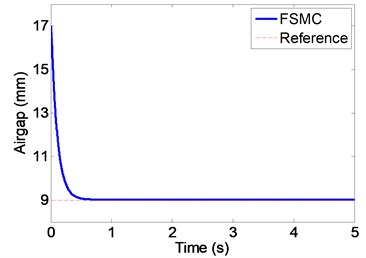Fig. 12Control current with FSMC (A)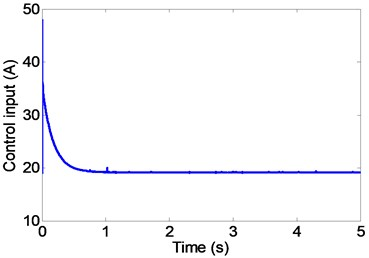Fig. 13Airgap under disturbance (FSMC)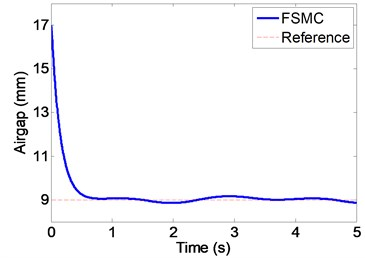Fig. 14Control current under disturbance (FSMC)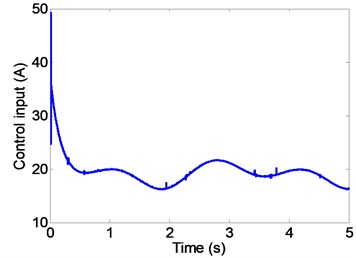Fig. 15The response of the airgap with multi-parameters perturbation (PID) (L varies by ± 20 %, m varies by ± 40 %, R varies by ± 20 %)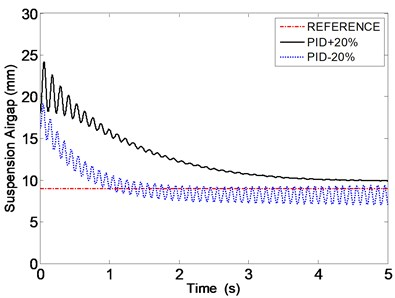To study the robustness of proposed control algorithm from the multi-parameters perturbations, the inductance also varies by ±20 %, the mass varies by ±40 %, and the resistance varies by ±20 %. The response of the airgap of the FSMC with multi-parameters perturbation is shown in Fig. 16. We can see that the FSMC can eliminate the mismatches between mathematical model and dynamics of the ELS with multi-parameters perturbations.

In conclusion, the simulation results indicate that the proposed controller using fuzzy inference method with sliding mode surface has strong robustness against the disturbance and multi-parameters perturbations, excellent dynamic and steady-state performance without chattering and high energy consumption.

Fig. 16The response of the airgap with multi-parameters perturbation (FSMC) (L varies by ±20 %, m varies by ±40 %, R varies by ±20 %)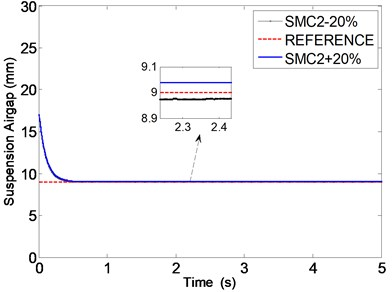## 4. Experimental results

After sufficient simulation tests, much effort has been put to implement experiments, with the aim of examining the practical performance of the fuzzy-sliding mode control scheme. The experiments are implemented in the national low-speed maglev test line. The full-scale test maglev train and maglev experimental line are shown in Fig. 17.

To fully investigate the performance of the proposed fuzzy-sliding mode controller (FSMC), two sets of experiments are implemented to evaluate the controller's tracking performance and the disturbance rejection capacity, respectively. In order to be compared, the experimental results of PID controller are also provided.

Fig. 17Train experiment placement in the test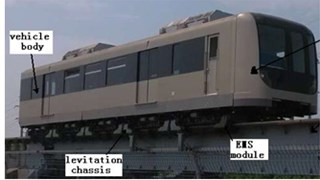a) Low-speed maglev train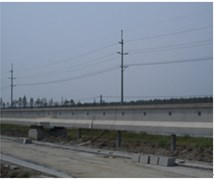b) Maglev experimental line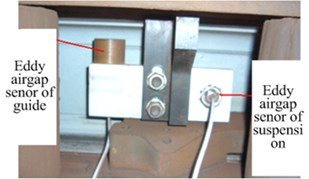c) Airgap sensor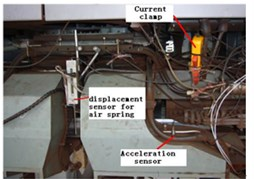d) Current sensor

### 4.1. Experiment 1: Levitating from stationary stat

The presented FSMC controller and PID controller are applied in the test train respectively. The maglev train is stationary on the rail during 0 s-14 s with the initial airgap of 17 mm. At 14 s, the train begins to be levitated to the target airgap of 9 mm. The dynamic response of the levitating airgap during the experiment is provided in Fig. 18.

The solid line in Fig. 18 represents the FSMC control performance and the dashed line denotes PID control performance. The first 14 s shows the train is on the rail and the initial airgap between rail and electromagnet is 17 mm. At 14 s, the train begins to track the target position. We can see from the Fig. 18 that the levitation airgap response of PID is fluctuant and the dynamic performance of FSMC is better, which can rapidly convergence to the target position. Moreover, The FSMC controller has no overshot. The control current response of PID and FSMC controller is illustrated in Fig. 19. We can see that though the FSMC is based on sliding mode control with chattering phenomenon in control inputs, the fuzzy control law has reduced the chattering effectively. The fluctuation of FSMC control current in Fig. 19 can meet the engineering application requirements.

Fig. 18Results of Experiment 1: Airgap (mm)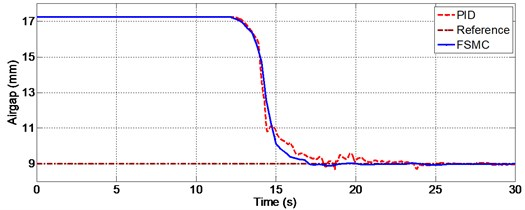Fig. 19Results of Experiment 1: Control current (A)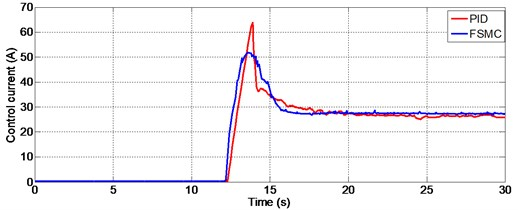### 4.2. Experiment 2: with periodic shock disturbance

When the trains arrive at station, the passengers get on or off the train will lead to inevitable shock disturbances to the levitating train. In this experiment, we take the jumping load of 10 people in the train as periodic shock disturbance. The airgap response results of the levitating train with conventional PID and proposed FSMC are provided in the Fig. 20 and Fig. 21, respectively. The experiment results show that the airgap with PID controller has large variational amplitude under the jumping disturbance, but the airgap with FSMC controller remains in the target position with small fluctuation. The acceleration of the train with FSMC is shown in Fig. 22. The response of the control current with FSMC is provided in Fig. 23. It is shown in the figure that the chattering phenomenon in control inputs is suppressed significantly, thus the FSMC can save energy consumption and reduce wear of the actuator.

Fig. 20Results of Experiment 2: Airgap with PID (mm)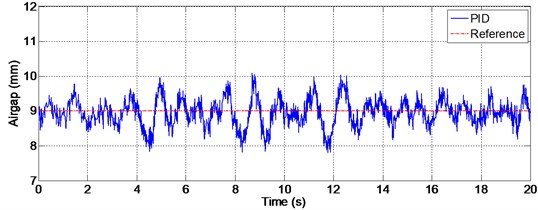Fig. 21Results of Experiment 2: Airgap with FSMC (mm)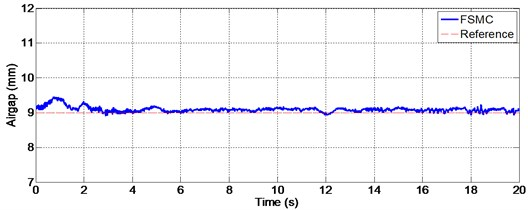Fig. 22Results of Experiment 2: Acceleration with FSMC (m/s2)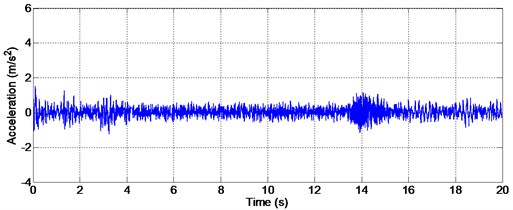Fig. 23Results of Experiment 2: Control current with FSMC (A)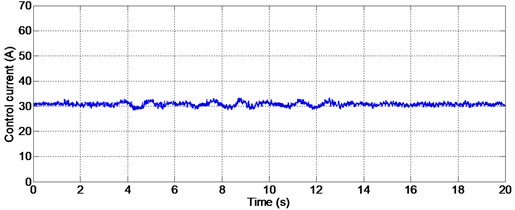The fuzzy sliding mode controller presented in this paper is more suitable for practice application in the maglev train. The experimental results of the test maglev train demonstrate that the FSMC control algorithm makes the train stable levitating on a target airgap with stronger robustness, better dynamic performance and higher anti-disturbance capacity.

## 5. Conclusions

The nonlinear dynamic model is constructed for the single-ELS. In order to improve the levitation dynamic performance of the maglev train, a fuzzy sliding mode controller is presented using fuzzy inference method with sliding mode surface to deal with disturbance, nonlinear and multi-parameters perturbation of the system. The simulation results illustrate that the proposed nonlinear controller has non-overshooting, strong robustness, fast dynamic response and less chattering, which has increased performance versus conventional PID controller. The chattering phenomenon of SMC can be reduced significantly by FSMC. The experimental results verify that the proposed controller can restrain the disturbance significantly with fast regulating speed and satisfy the control quality request of the low-speed maglev train.

#### Cited by

Artificial Intelligence
Robust nonsingular sliding mode control of the maglev train system: case study
Nonlinear dynamic characteristic modeling and adaptive control of low speed maglev train
2020 39th Chinese Control Conference (CCC)
Adaptive levitation control for characteristic model of low speed maglev vehicle
2019 IEEE 23rd International Conference on Intelligent Engineering Systems (INES)
Dynamic Modeling and Adaptive Sliding Mode Control for a Maglev Train System Based on a Magnetic Flux Observer

02 August 2016
Accepted
02 January 2017
Published
15 February 2017
SUBJECTS
Vibration generation and control
Keywords
electromagnet levitation system
nonlinear dynamics
sliding mode control
fuzzy control
Acknowledgements

This research is supported by Key Projects in the National Science and Technology Pillar Program of China (2013BAG19B00-01) and the National Natural Science Foundation of China (No. 51505277).

Author Contributions

Sun Yougang contributed significantly to analysis and manuscript preparation; Li Wanli contributed to the conception of the study. Xu Junqi performed the experiments. Qiang Haiyan performed the data analyses and wrote the manuscript; Chen Chen helped perform the analysis with constructive discussions.